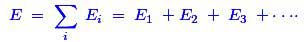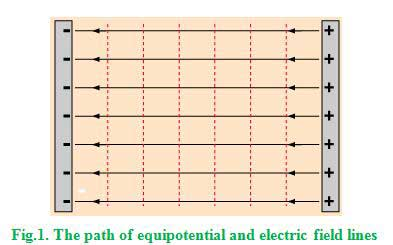# MCQs on Electric Circuits

##### Page 18 of 63. Go to page 1 2 3 4 5 6 7 8 9 10 11 12 13 14 15 16 17 18 19 20 21 22 23 24 25 26 27 28 29 30 31 32 33 34 35 36 37 38 39 40 41 42 43 44 45 46 47 48 49 50 51 52 53 54 55 56 57 58 59 60 61 62 63
01․ If two point charges are denoted by + Q and - Q and distance between this charges is d, then the dipole moment P equal to
Q2 × d.
Q × d.
Q / d2.
Q2 / d.

If an electric dipole has two point charges +Q and –Q separated by the distance d, then the dipole moment P would be the product of the charge and the distance.

02․ Electric displacement is a ____________________ quantity.
scalar
vector
both of above
none of above.

The electrical displacement represents the component of an electric field associated solely with the presence of separated free electric charges, purposely excluding the contribution of any bound electric charges in neutral atoms or molecules. Hence, that should be a vector quantity.

03․ A unit tube of flux is known as ____________________ tube.
Newton
Michale
none of above.

Faraday is a famous physicist who expressed the principle of tube of flux. That is why his name became the unit of tube of flux.

04․ The unit of electric displacement vector is
C/m2.
C/m.
Ampere-m.
C - m.

The electric displacement field is referred as the movement of charge through unit area. Hence, the unit is C/m2.

05․ Electric field intensity ( E ) at any point in an electric field is equal to

The total electric field at any point is equal to the vector sum of the separate electric fields that each point charge would create in the absence of the others. That is,The electric field is nothing but the potential gradient of that particular point.

06․ An electric field line and an equipotential surface are
always perpendicular.
always parallel.
inclined at any angle.
none of above.

For parallel conducting plates like those in a capacitor, the electric field lines are perpendicular to the plates hence they are parallel to each other and the equipotential lines are parallel to the plates.07․ Which of following is not a vector?
Linear momentum.
Angular momentum.
Electric field.
Electric potential.

An electric field intensity E is related with the electric potential φ through the expression E = − ∇φ. Gradient of a scalar electric potential is a vector electric field intensity.

08․ The ratio of electric flux density to electric field intensity is called ......... of medium
permeability
permittivity
reluctance
capacitance

From the application of the Gauss law, ε = D / E Where, D is electric flux density and E is electric field intensity.

09․ The value of E within the field due to a point charge can be found with help of
Kirchhoffs law.
Lenzs law.
Coulombs law.

Coulomb’s law is the law which deals with the electric charge directly. The Faraday’s Law and Len'z Law deals with electromagnetic induced emf and Kirchhoff’s Law is deals with currents and voltage in electric circuits.

10․ To prevent the generation of static charges on rubber or flat leather
surface is moistened.
conductive dressing is done.
oil compound dressing is done.
talcum powder is sprayed on the surface.

Static electricity is caused by the movement of electrons when two dissimilar substances are separated. But, the conductive dressing system would not allow them to separate with each other.

<<<1617181920>>>